# Part 3: Trigonometric Ratios | Free Worksheet

In this article, we walk you through trigonometric ratios. We explain the rules and show you how to apply them.## Year 10 Trigonometric Ratios

In this article, we’re going to discuss Year 10 trigonometric ratios. First, we’ll explain the theory and rules for trigonometric ratios. Then we’ll work through some questions with you. Finally, we’ve given you some concept check questions where you can test your knowledge and check your answers against our worked solutions.

## Syllabus Outcomes

Year 10 Trigonometric Ratios has the following outcomes for students to meet:

• Applies trigonometry, given diagrams, to solve problems, including problems involving angles of elevation and depression
• Applies trigonometry to solve problems, including problems involving bearings
• Applies Pythagoras’ theorem, trigonometric relationships, the sine rule, the cosine rule and the area rule to solve problems, including problems involving three dimensions

So, what do these mean for you? Remember when you did Trigonometry in Year 9? In this unit, you will build on that knowledge and learn how to apply this to solve real-life problems.

## Outline of Year 10 Trigonometric Ratios

Trigonometry, which comes from the Ancient Greek words trigōnon (triangle) and metron (measure), is exactly that: the tools which we use to measure triangles.

This subject guide will focus on Trigonometric Ratios directly, and talk about what the sine, cosine and tangent ratios mean and how to use them.

## Assumed Knowledge

Before reading this guide, we assume you have got a firm grasp of:

• Algebraic techniques
• Function notation
• Properties of right triangles (the hypotenuse, and Pythagoras’ theorem).

## Contents

In this guide, we will cover:

• A review of the three fundamental trigonometric ratios: sine, cosine and tangent;
• The reciprocal ratios secant, cosecant and cotangent;
• Exact trigonometric ratios;
• Related angles and trigonometric ratios;
• Trigonometric ratios for angles in other quadrants;
• Solving trigonometric equations.

For more trigonometric applications including graphing and word problems, see our other subject guide: Further trigonometry (Applications).

## Want to see how well you know Trigonometric ratios## Summary

A trigonometric function (for example sin, cos, tan) takes an ANGLE and gives you the corresponding RATIO of lengths in a right-angled triangle.

Below is a table of what each ratio corresponds to in a right triangle: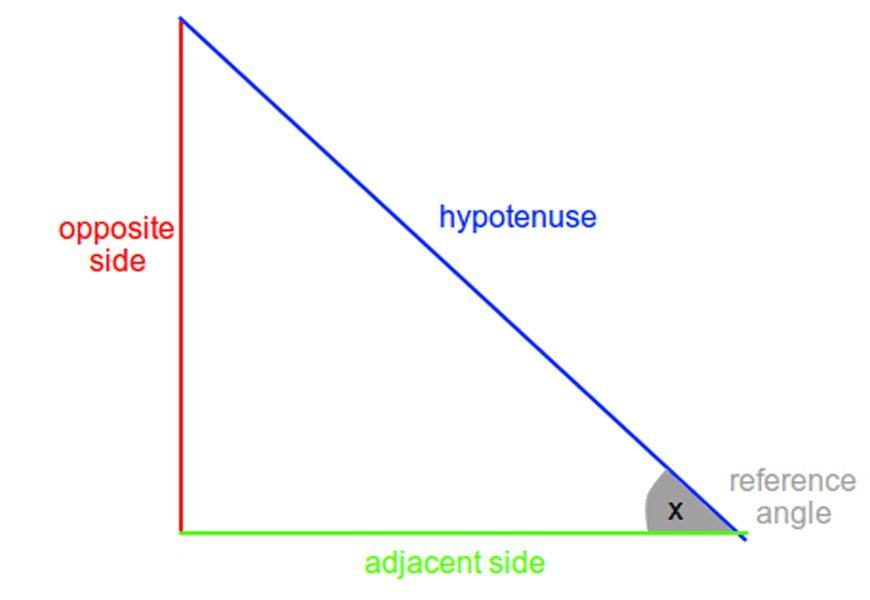$$\sin(x) :$$ $$\frac{\text{Opposite}}{\text{Hypotenuse}}$$ $$\cos(x):$$ $$\frac{\text{Adjacent}}{\text{Hypotenuse}}$$ $$\tan(x):$$ $$\frac{\text{Opposite}}{\text{Adjacent}}$$ $$\text{cosec}(x):$$ $$\frac{\text{Hypotenuse}}{\text{Opposite}}$$ $$\sec(x):$$ $$\frac{\text{Hypotenuse}}{\text{Adjacent}}$$ $$\cot(x):$$ $$\frac{\text{Adjacent}}{\text{Opposite}}$$

The ratios in the second row are known as “reciprocal ratios”, because they are the reciprocals of the corresponding ratio in the first row.

In addition to the reciprocal ratios, the trigonometric ratios are linked by their complementary angles.

A table of these relations is available below:

 $$\sin(x):$$ $$\cos(90-x)$$ $$\cos(x):$$ $$\sin(90-x)$$ $$\tan(x):$$ $$\cot(90-x)$$ $$\text{cosec}(x):$$ $$\sec(90-x)$$ $$\sec(x):$$ $$\text{cosec}(90-x)$$ $$\cot(x):$$ $$\tan(90-x)$$

## Trigonometric ratios

A number of common trigonometric ratios which appear in high school are listed below:

### 30-60-90 triangle:

• $$\sin 30 = \frac{1}{2}$$
• $$\cos 30 = \frac{\sqrt{3}}{2}$$
• $$\tan 30 = \frac{1}{\sqrt{3}}$$
• $$\sin 60 = \frac{\sqrt{3}}{2}$$
• $$\cos 60 = \frac{1}{2}$$
• $$\tan 60 = \sqrt{3}$$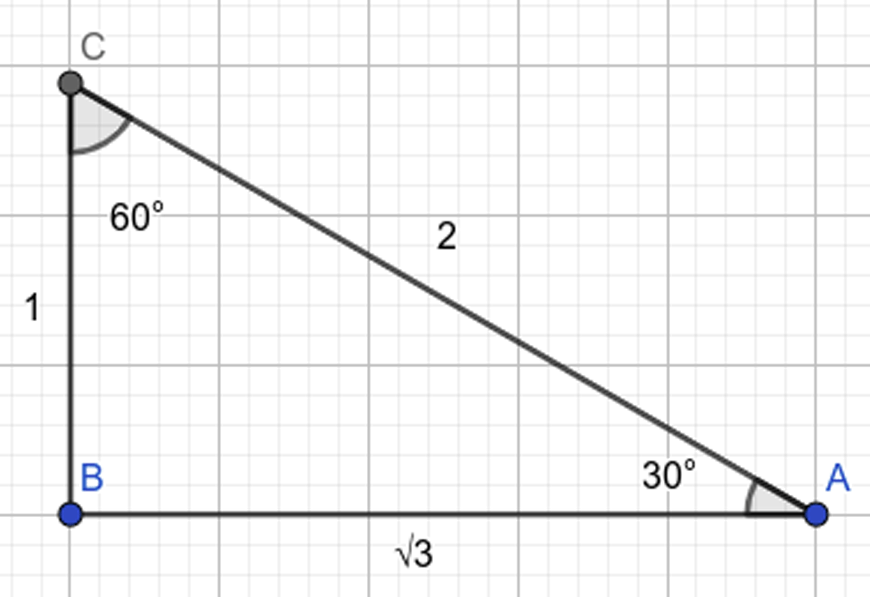### 45-45-90 (right isosceles triangle):

• $$\sin 45 = \frac{1}{\sqrt{2}}$$
• $$\cos 45 = \frac{1}{\sqrt{2}}$$
• $$\tan 45 = 1$$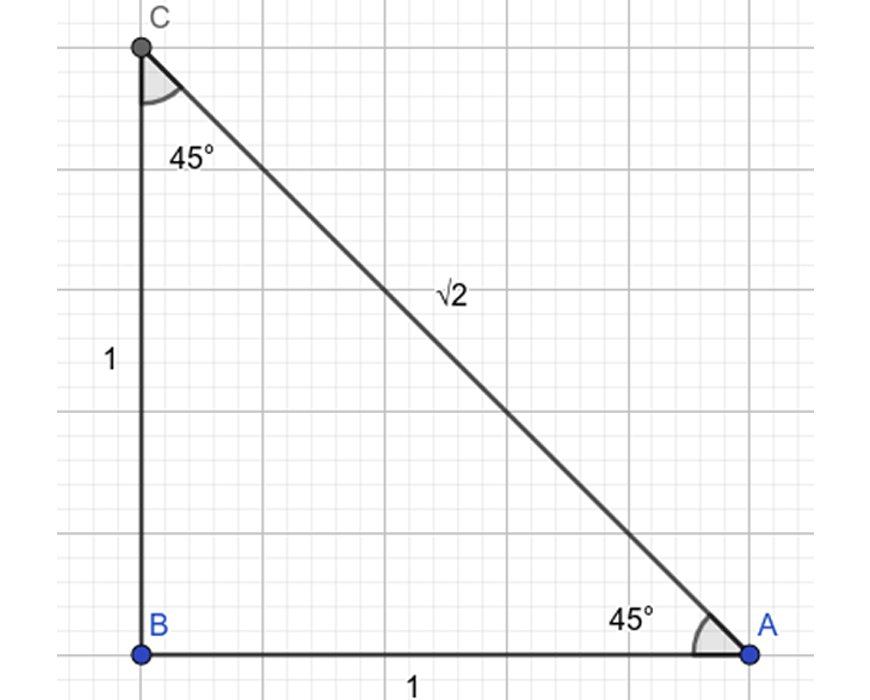Each base angle in the range $$0° < x < 90°$$ has many related angles, which can lie in different quadrants.

Except for the sign (positive or negative), the trigonometric ratio of a larger angle is the same as the trigonometric ratio of its related base angle.

## Related Ratios:

• $$\sin 140° = \sin (180-40)° = \sin 40°$$
• $$\tan 220° = \tan (220-180)° = \tan 40°$$
• $$\cos 320° = \cos (360-40)° = \cos 40°$$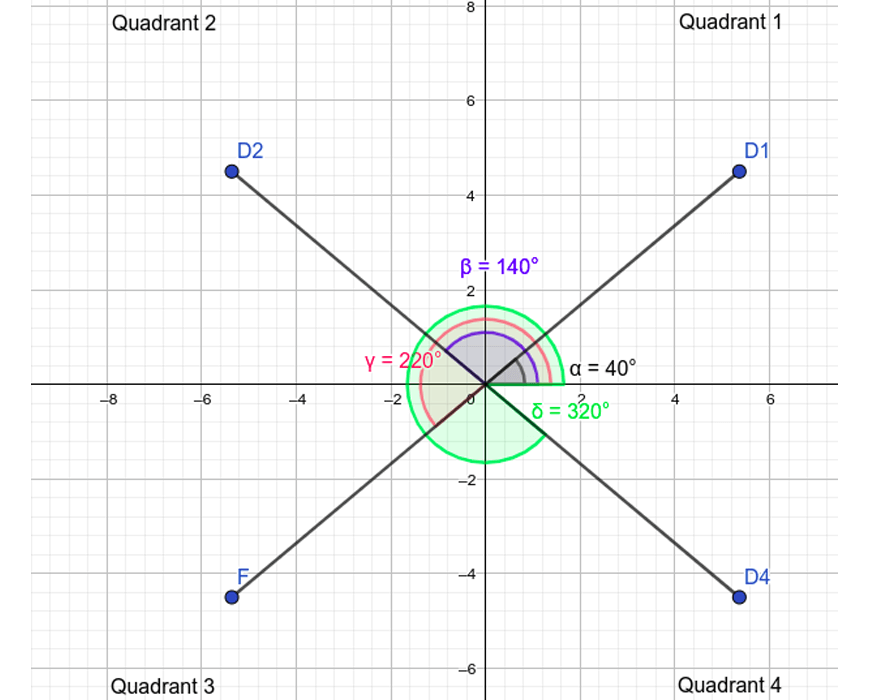To find the base angle of a related angle, try adding or subtracting multiples of $$360°$$ until the angle is in the range $$0° -360°$$.

Then use the above transformations $$(180-x°, 180+x°, 360-x° )$$ to get the angle into the first quadrant.

When calculating trigonometric ratios, the quadrant which the angle lies in affects the sign of the ratio.

The mnemonic ALL STATIONS TO CENTRAL can be used to remember which ratios are positive in the given quadrants: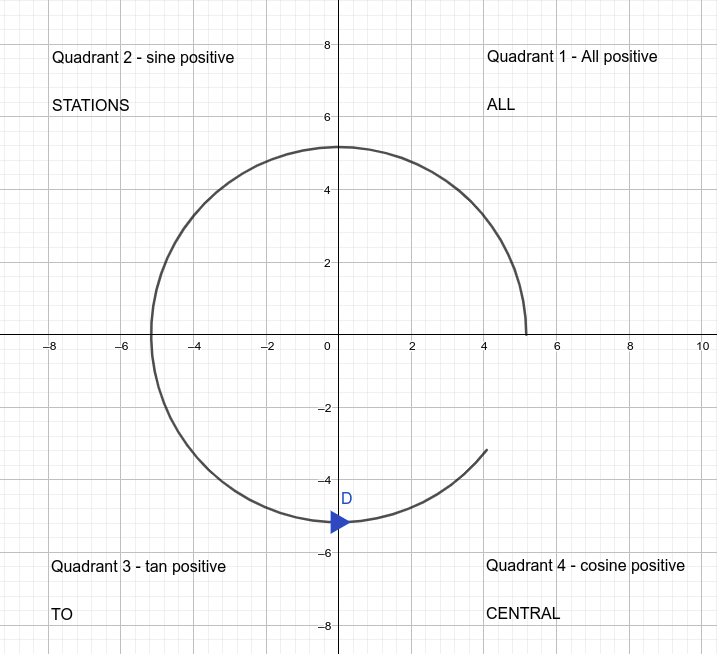To solve a trigonometric equation, the inverse trigonometric functions are available on your calculator (in yellow, above the regular $$\sin, \cos \text{and} \tan$$ keys).

A question might ask to to solve for $$x$$, given that $$\sin x = 0.7$$.The solution would then be $$x=\sin^{-1} 0.7 = 44°$$ (to the nearest degree).

## Concept Check questions

1. Given that the trigonometric ratio $$\sin x = 7\over10$$ and $$x$$ is in the first quadrant, find the exact value of the following expressions:

a) $$\text{cosec} x$$

b) $$\cos x$$

2. Find $$x$$, if $$0° <x<180°$$:

a) $$\cos x = \frac{1}{2}$$

b) $$\tan x = 0.6$$

c) $$\sin x = 0.4$$

3. Express the following ratios in exact form.

a) $$\sin 30°$$

b) $$\cos 300°$$

c) $$\tan -585°$$

## Solutions

1.

a) Since $$\text{cosec} x$$ is the reciprocal of $$\sin x$$, $$\text{cosec} x = \frac{1}{\frac{7}{10}} = \frac{10}{7}$$.

b) To do this question, we need to draw a triangle: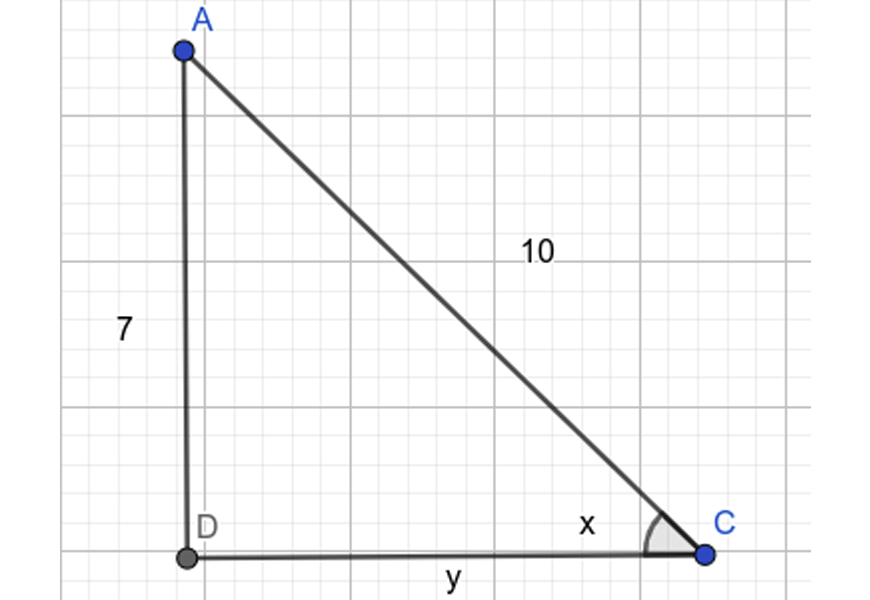For this triangle, we know the ratio of 2 sides from $$\sin x$$. To find the other side, we can use Pythagoras’ theorem:

$$y^2=10^2-7^2=51$$

Hence

$$y=\sqrt {51}$$

Then, since $$\cos$$ is adjacent over hypotenuse,

$$\cos x = \frac{\sqrt{51}}{10}$$

2.

a. This is an exact trigonometric ratio, as you should recall, so $$x=60°$$

b. Using the $$\tan^{-1}$$button on our calculator, we have:

$$x = \tan^{-1} 0.6 = 31°$$ (nearest degree).

c. Using the $$\sin^{-1}$$ button on our calculator, we have

$$x = \sin^{-1} 0.4 = 24°$$ (nearest degree)

However, in the range $$0° < x < 180°$$

there is another solution:

$$x = (180 – \sin^{-1} 0.4 )° = 156°$$

Hence:

$$x = 24° \text{or} 156°$$

3.

a) From our list, we know that $$\sin 30° = \frac{1}{2}$$

b) The angle lies in the 4th quadrant; and $$\cos$$ is positive in the 4th quadrant.

The related angle is $$360 – 300 = 60°$$, so $$\cos 300° = \frac{1}{2}$$

c) First, we add 2 multiples of 360 to get the angle into the range $$0°<x<360°$$:

$$\tan (-585 + 720)° = \tan 135°$$

Then we note that it’s in the 2nd quadrant, making it negative for tan.

Then we apply the transformation $$180-135 = 45°$$ to get it into the first quadrant. Since $$\tan 45° = 1$$,

we have $$\tan -585° = -1$$.

## Additional Challenging Questions (From the MAX Series)

### Questions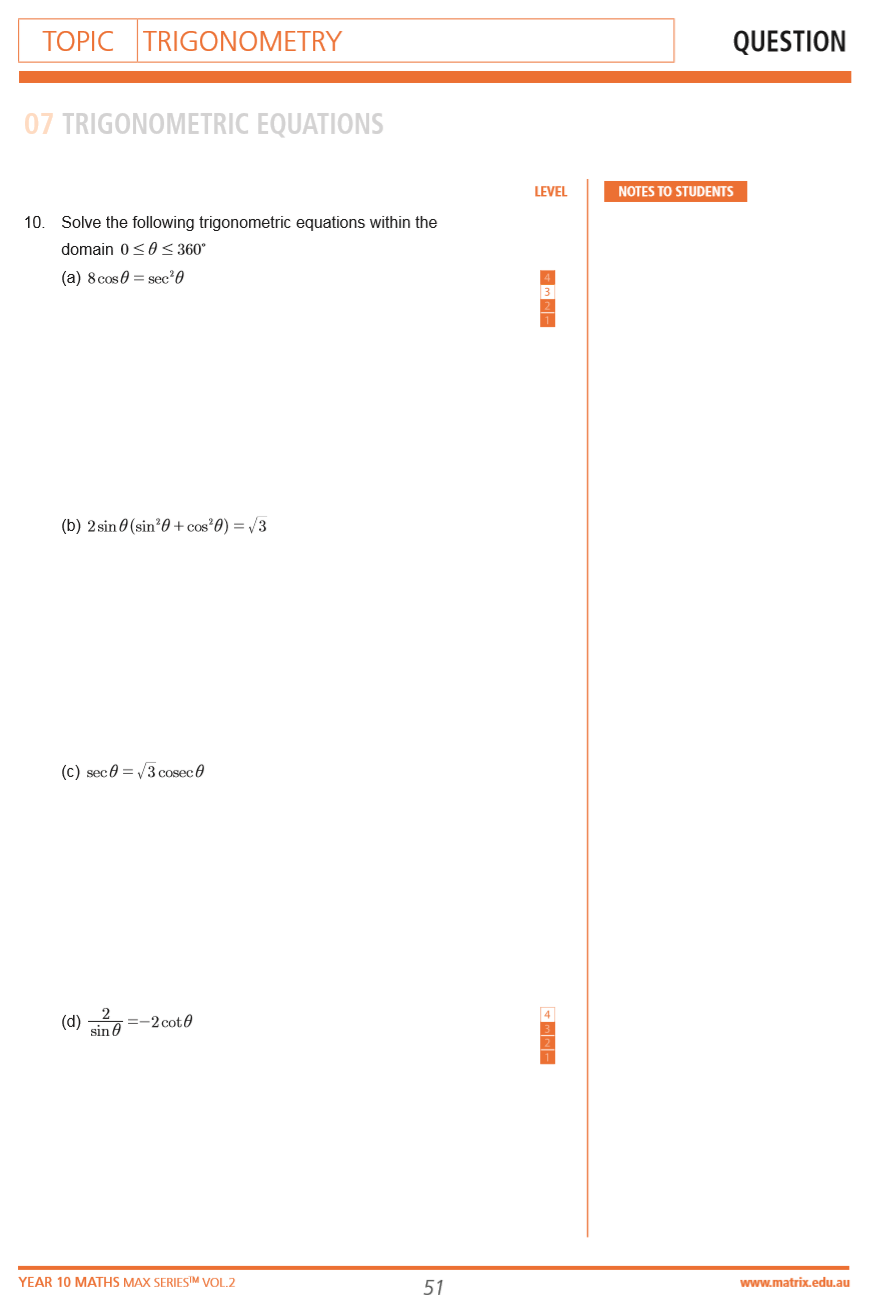Questions from the Year 10 MAX Series Vol. 2 Trigonometry

### Solutions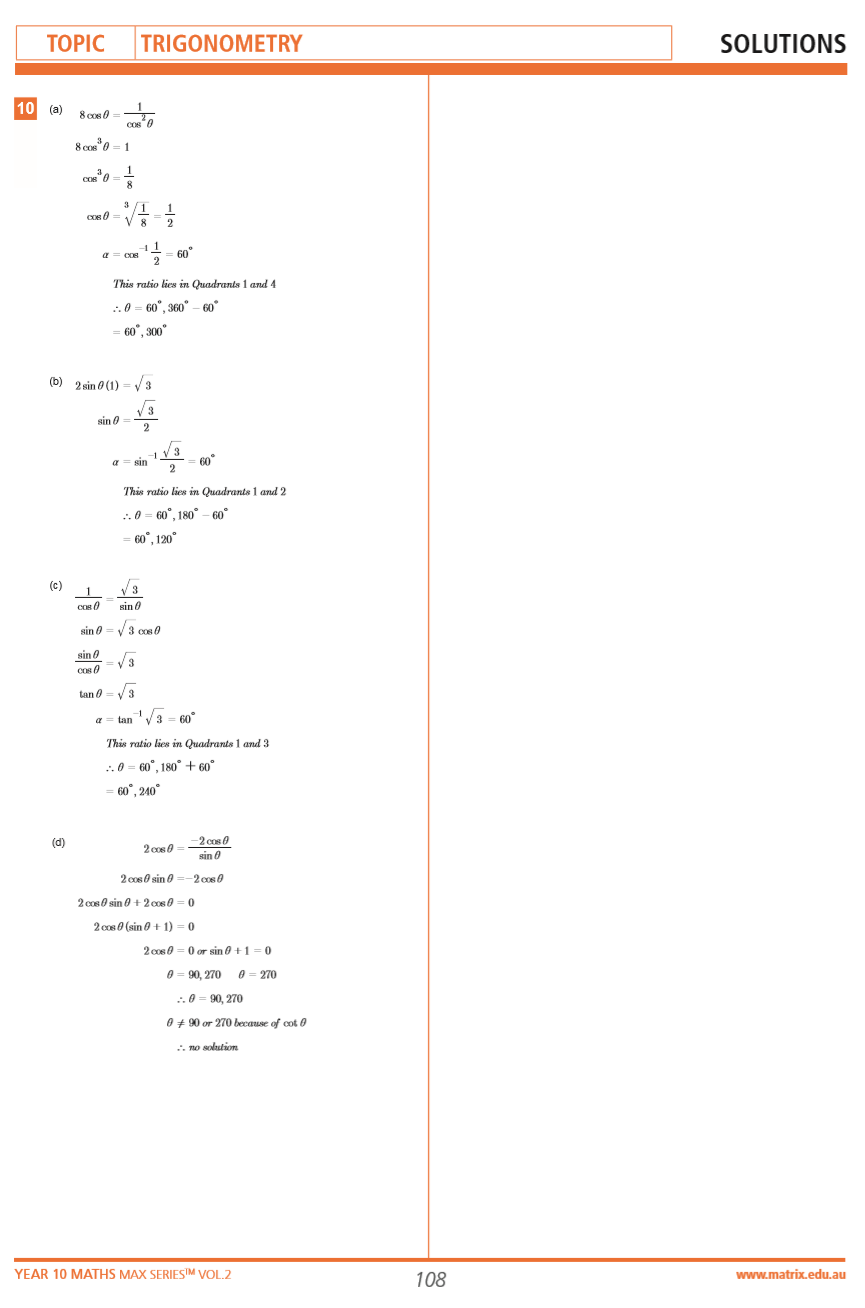Solutions from the Year 10 MAX Series Vol. 2 Trigonometry

## Understand how to use trigonometric ratios

In our next article, we show you the practical applications of what you’ve learned in trigonometry so far.

© Matrix Education and www.matrix.edu.au, 2021. Unauthorised use and/or duplication of this material without express and written permission from this site’s author and/or owner is strictly prohibited. Excerpts and links may be used, provided that full and clear credit is given to Matrix Education and www.matrix.edu.au with appropriate and specific direction to the original content.